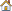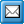﻿ Constants
QPR Knowledge Base 2017.1

ConstantsMetrics User's Guide > Using QPR Metrics > Formulas:

ConstantsConstantsFormulas can contain the following constants:

Type

Format

Examples

Integer

[+|-]<n>

123, -311, +3

Floating Point

[+|-]<n>[.<n>]

0.45, -31.1, +0.1

Scientific

[+|-]<n>[.<n>]E[+|-]<n>

11E1, -1.13E3, +51E2, 0.3421E-5

Boolean

FALSE|TRUE

FALSE, TRUE

Text

"<t>"

"Lorem ipsum"

Used markings:

 numeric value [ ] optional part text value

Note that the decimal separator for the floating point and the scientific numbers is not dependent on Windows' Regional Options. The separator is always a point (".") regardless of the regional settings.

Note that to use a double quotation mark as a part of a text constant, you need to precede the double quotation mark with a backslash. For example, a text constant defined as "ab\"c" will have the value ab"c.

Scientific notation was designed for easy representation of floating point numbers that are either very large or very small. A scientific number consists of base and exponent which are separated from each other with a letter E: <base>E<exponent>. The exponent is the exponent of 10. To calculate the real value of a scientific number, use the following formula: <base>*10<exponent>.